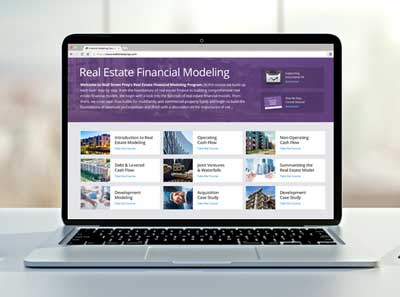Welcome to Wall Street Prep! Use code at checkout for 15% off.# Cap Rate

Quick Primer on Understanding the Capitalization Rate in Real Estate (2023 Update)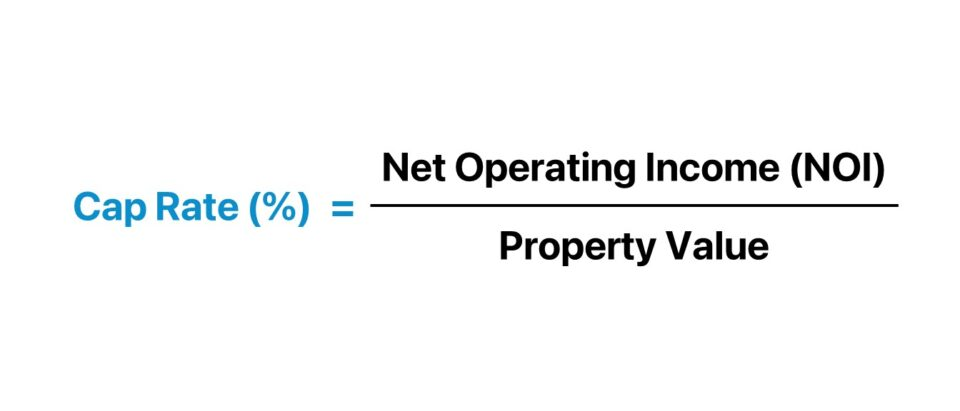• The cap rate is the potential return rate on a rental property, such as a commercial building, based on its income potential and risk.
• The cap rate formula divides the net operating income (NOI) of a rental property by the market value of the property as of the present date.
• The higher the cap rate, the higher the risk and the potential return – all else being equal.
• There is no good cap rate, per se, because the decision is subjective to a specific investor, but most commercial real estate (CRE) investors perceive a cap rate between 4% and 10% as favorable.

## How to Calculate Cap Rate?

The cap rate is the primary shorthand by which different properties with comparable risk-return profiles can be analyzed side-by-side.

The cap rate in real estate is a fundamental return metric that compares the annual net operating income (NOI) of a property to its current market value to measure the expected yield.

In practice, the participants in the commercial real estate (CRE) market closely analyze potential investment opportunities to determine an appropriate purchase price at which the target return is attainable.

The cap rate is calculated by dividing the net operating income (NOI) of an investment property by its market value as of the present date.

• Net Operating Income (NOI) → The NOI measures the income potential of a property based on its core drivers of revenue, such as rent payments, minus its operating expenses, e.g. maintenance costs, property taxes, and insurance. However, the NOI used to analyze the capitalization rates of rental properties must be stabilized to reflect their “steady-state” performance.
• Property Value → The current market value, on the other hand, is the property’s fair market value (FMV) as of the present date. The fair value is determined in an independent appraisal and is intended to reflect the current value of the property, rather than the original purchase price.

By converting the NOI into a percentage, the cap rate is a standardized metric that facilitates “apples-to-apples” comparative analysis between a peer group of comparable properties to determine the investment that offers the most attractive risk-return trade-off.

Based on the cap rate, a real estate investor can also derive the payback period, the estimated number of years needed for the investment property to pay for itself (and recoup the original capital contribution).

The steps to calculate the cap rate are as follows:

• Step 1 → Calculate the Net Operating Income (NOI) of the Property at Stabilization
• Step 2 → Determine the Market Value of the Property as of the Present Date
• Step 3 → Divide the Annual NOI of the Property by its Current Market Value
• Step 4 → Multiply by 100 to Convert from Decimal Notation to Percentage Form## Cap Rate Formula

The formula used to calculate the cap rate is the net operating income (NOI) of the property divided by its present market value.

Cap Rate (%) = Net Operating Income (NOI) ÷ Property Value

The NOI of the properties must be expressed on a pro forma basis to reflect the income that the properties expect to generate at stabilization, which refers to the state at which a real estate development project or acquisition is near complete, with the property near full occupancy in leasing and rent prices close to the market rate.

The formula used to calculate net operating income (NOI) is the sum of rental and ancillary income, less direct operating expenses.

• Net Operating Income (NOI) = (Rental Income + Ancillary Income) – Direct Operating Expenses

The NOI can also be computed by subtracting a property’s effective gross income (EGI) from its direct operating expenses.

• Potential Gross Income (PGI) = (Total Number of Units × Annual Market Rate Rent) + Other Income
• Effective Gross Income (EGI) = Potential Gross Income (PGI) – Vacancy and Credit Losses
• Net Operating Income (NOI) = Effective Gross Income (EGI) – Direct Operating Expenses

Note: The property value refers to the current market value of the property as of the present date, i.e. the fair value. The purchase cost (or asking price) can also be used depending on the context of the analysis.

###### Does Cap Rate Include Mortgage Payments?

One of the more common questions regarding the capitalization rate received by students and trainees is, “Does the cap rate formula include mortgage payments?”

The answer? No, the cap rate calculation does not include mortgage payments or interest on real estate loans.

The rationale for the capitalization rate metric neglecting mortgage and interest payments is that those spending activities are categorized as financing costs rather than operating costs.

Cap rates are therefore an unlevered measure of returns used to analyze individual investment properties and perform comps analysis.

• Financing Structure → The funding sources of the real estate project are at the discretion of the new investor post-closing, so prior capitalization does not matter, for the most part.
• Unlevered Return Metric→ Since the effects of debt financing are neglected in net operating income (NOI) – unlike financial metrics such as net income – the cap rate is an “unlevered” measure of returns suited for comparability.

## Cap Rate Calculator

We’ll now move on to a modeling exercise, which you can access by filling out the form below.#### Get the Excel Template!Submitting...

## 1. Commercial Real Estate Building Assumptions

Suppose a commercial real estate investment firm (CRE) is analyzing a potential rental property investment opportunity with a current property value of \$75 million.

The pro forma financial data for the commercial real estate investment – the strategic acquisition of an office building – for the fiscal year ending 2023 is as follows.

Commercial Office Building – Financial Data:

• Potential Gross Income (PGI) = \$10.2 million
• Vacancy and Credit Losses = (\$200k)
• Property Management Fees = (\$1,000k)
• Maintenance and Repairs = (\$500k)
• Property Taxes = (\$800k)
• Property Insurance = (\$600k)

## 2. Net Operating Income (NOI) Calculation Example

The CRE investment firm decides a good starting point is to estimate the property’s cap rate, in which the first step is to compute the property’s effective gross income (EGI).

The difference between potential gross income (PGI) and vacancy & credit losses is the effective gross income (EGI) of the commercial property, which amounts to \$10 million.

• Effective Gross Income (EGI) = \$10.2 million – \$200k = \$10 million

In the next step, we’ll deduct the operating expenses of the commercial real estate property to arrive at the property’s net operating income (NOI), which is \$7.1 million.

• Net Operating Income (NOI) = \$10 million – \$1 million – \$500k – \$800k – \$600k = \$7.1 million

Therefore, the commercial office building is expected to generate \$7.1 million in stabilized NOI.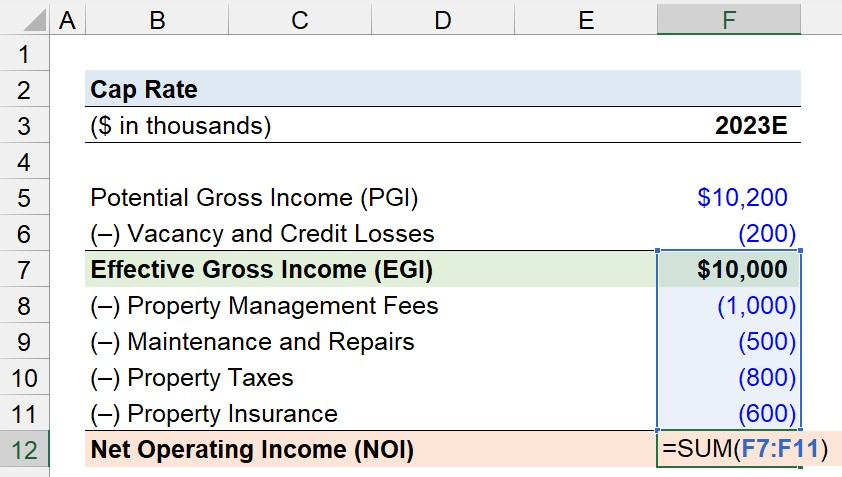## 3. Cap Rate Calculation Example

Since the property value was presented as an assumption earlier, whereas the NOI was just calculated in the prior section, the required formula inputs to compute the capitalization rate are set.

• Net Operating Income (NOI) = \$7.1 million
• Property Value = \$75 million

By dividing the net operating income (NOI) of the commercial real estate property by the current property value, we arrive at an implied cap rate of 9.5%.

• Cap Rate (%) = \$7.1 million ÷ \$75 million = 9.5%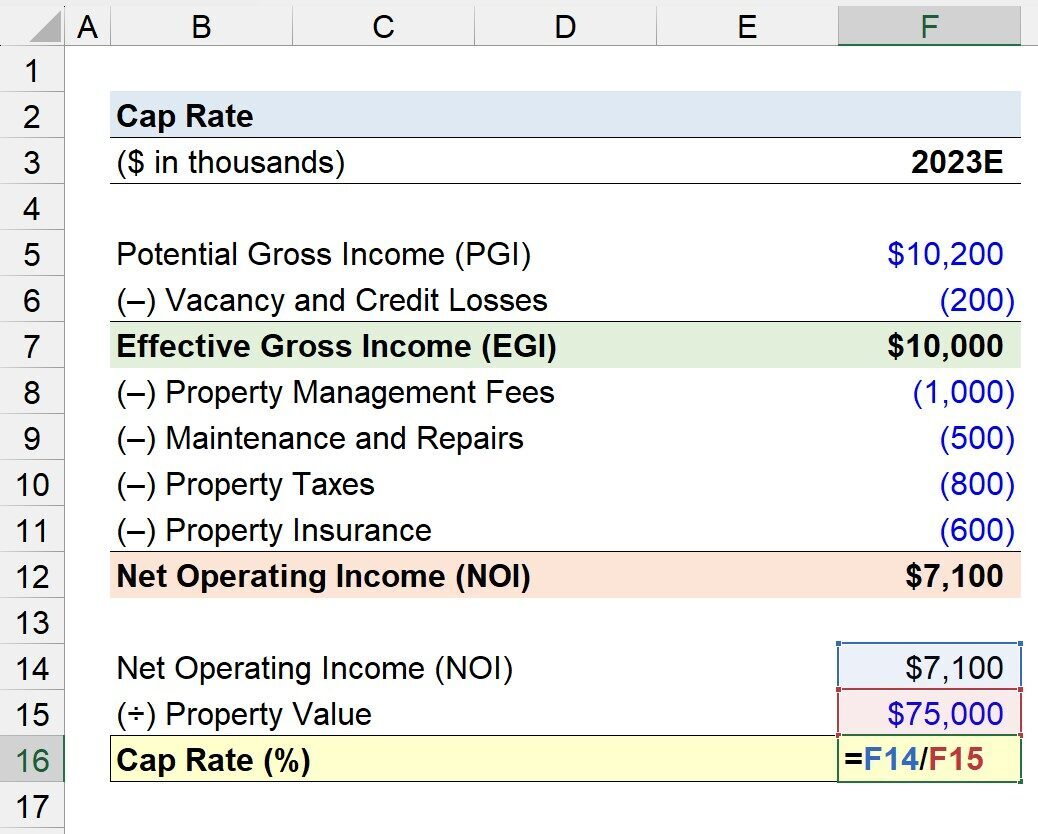## 4. Cap Rate Valuation Analysis Example

In the final part of our modeling exercise, we’ll solve the estimated property value of the commercial real estate (CRE) property using the direct capitalization method.

The property value of the commercial real estate (CRE) office building was previously stated as an assumption, but we can neglect that, for now, to better illustrate the relationship between NOI and capitalization rate.

The annual NOI we computed is \$7.1 million, while the capitalization rate was 9.5% (or, more specifically, 9.467%).

Upon dividing our property’s net operating income (NOI) by its cap rate, we arrive at an implied property value of \$75 million, which matches the original assumption stated at the start of our tutorial.

• Implied Property Value = \$7.1 million ÷ 9.467% = \$75 million## NOI / Cap Rate Formula

Under the income approach, or “direct capitalization method,” the value of a real estate investment property is estimated by dividing the property’s net operating income (NOI) by its cap rate.

Implied Property Value = Net Operating Income (NOI) ÷ Market Cap Rate

For example, suppose a commercial office building is expected to generate \$12 million in net operating income (NOI) in the coming year.

Comparable properties located nearby currently trade at a 7.5% cap rate based on analyzing the market and location-specific factors.

What does a 7.5% cap rate mean?

A 7.5% cap rate implies that the rental property’s net operating income (NOI) equals 7.5% of its market value.

Hence, a property with a value of \$12 million and a 7.5% cap rate exhibits an annual return of \$900k.

• Annual Return (%) = \$12 million × 7.5% = \$900k

One property appraisal method to determine the estimated valuation of the property is to use the capitalization rate derived from the peer group to guide the pricing analysis.

Given the 7.5% cap rate, the pricing of our property investment is implied to be approximately \$160 million.

• Property Price = \$12 million ÷ 7.5% = \$160 million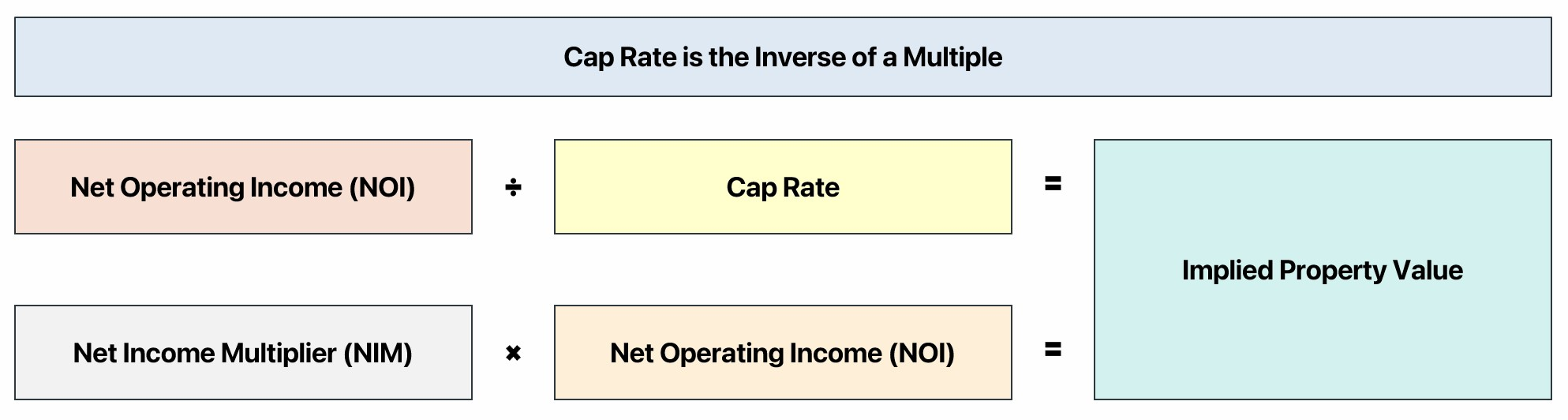“Cap Rate is the Inverse of a Multiple”

## What is a Good Cap Rate for a Rental Property?

The cap rate is a measure of returns, so the metric is also a measure of risk, since risk and return are two sides of the same coin.

So, is it better to have a higher or lower cap rate?

In short, the answer is rather nuanced and entirely dependent on the investor (and surrounding circumstances).

Contrary to a common misconception, a higher cap rate is not always preferred by real estate investors.

The reason? Higher cap rates are often achieved by investing in riskier properties, so the trade-off between risk and return must be understood.

A higher cap rate implies more risk attributable to a real estate property investment (and vice versa for a lower cap rate).

Certain real estate investors prioritize capital preservation – i.e., minimizing the risk of capital loss on an investment – whereas others are more yield-oriented and set a higher bar for the required rate of return.

The risk tolerance varies by the investor, which goes hand-in-hand with the prior factor, as more risk should correspond with higher returns to compensate the investor for undertaking the incremental risk (and vice versa).

For instance, a risk-averse real estate investor pursuing a long-term, steady stream of income is likely to prefer properties with lower capitalization rates located in stable markets, instead of riskier properties with higher cap rates.

• Higher Cap Rate → An investment property with a higher cap rate is perceived as a riskier investment with less stability in its cash flow generation. However, the increased risk attributable to the property causes the investment to be more appealing to yield-oriented investors, as the potential to reap more profits and greater returns is higher.
• Lower Cap Rate → On the other hand, a property with a lower cap rate is viewed as a safer investment with a more stable performance in cash flow production. The drawback to the predictable stream of net operating income (NOI) is that the return is also foreseeable, barring unusual circumstances.

## Cap Rate vs. Yield: What is the Difference?

The difference between the cap rate and cash yield (or “cash-on-cash return”) is as follows.

• Cap Rate → The cap rate is the return expected on a rental property investment. Contrary to the cash yield, the cap rate neglects the effects of financing (i.e. capital structure neutral), since net operating income (NOI) is the numerator. NOI is an unlevered profit metric unaffected by discretionary financing decisions. Hence, the cap rate excludes financing costs such as interest and mortgage payments.
• Cash Yield → The cash yield, or “cash on cash return”, is the annual pre-tax levered cash flow received per dollar of equity invested. Unlike the cap rate, the cash yield is a levered metric (post-financing) because the annual pre-tax cash flow is deducted by the annual debt service, which includes interest and principal payments.

The formula to calculate the cash yield comprises dividing the annual levered pre-tax cash flow of a property by the equity investment contributed by the real estate investor.

Cash Yield (%) = Levered Pre-Tax Cash Flow ÷ Equity Contribution

The other distinction between the cap rate and cash yield – aside from the unlevered and levered numerator – boils down to the denominator.

The constant denominator in the cash yield metric remains indifferent to the current state of the real estate market and economic conditions, since the purchase price (and equity contribution) is fixed.

Therefore, the cap rate is a pro forma metric that measures the implied return on an investment property, while the cash yield determines the return earned on an equity investment on a rolling basis.

## Cap Rate Compression vs. Expansion: What is the Difference?

If the cap rates in a particular real estate market decline, the market is said to be in a state of “cap rate compression.” Conversely, if cap rates rise, the market is undergoing “cap rate expansion.”

As a general rule of thumb, a real estate market experiencing cap rate compression should be expected to observe rising property asset values – all else being equal.

Why? The cap rates on rental properties and property values are inversely related.

• Cap Rate Compression → Increase in Property Values (and Rise in Purchase Prices)
• Cap Rate Expansion → Decrease in Property Values (and Decline in Purchase Prices)

Contrary to a frequent misconception, lower cap rates do not simply mean less risk in a potential investment property.

While the statement can be true in certain scenarios, there are exceptions in which the market cap rate declines following a widespread increase in property asset values.

Inflated property valuations in irrational markets often coincide with market factors that contribute to lower returns in the real estate asset class.

• Higher Purchase Price → A real estate market characterized by high purchase prices creates an unfavorable environment for new investors, where there is a higher risk of overpaying.
• Increased Market Demand → An increase in competition between buyers and investors causes property valuations to rise, often to an unreasonable level (i.e. seller’s market) – all else being equal. Therefore, the purchase price paid by the investor or buyer likely contains a significant purchase premium, often termed the “winner’s curse”.
• Cyclicality Risk → An investor that acquires a property at “peak” valuations in a cyclical market to opportunistically profit from short-term trends can backfire and incur steep losses.

The scenario in which cap rate compression is unfavorable to investors stems from a rise in property values without a proportionate increase in net operating income (NOI).

If the net operating income (NOI) of properties in the market increases at a comparable pace (or perhaps outpaces) the observed rise in property values, the investment opportunities are far more likely to be profitable and generate strong, positive returns.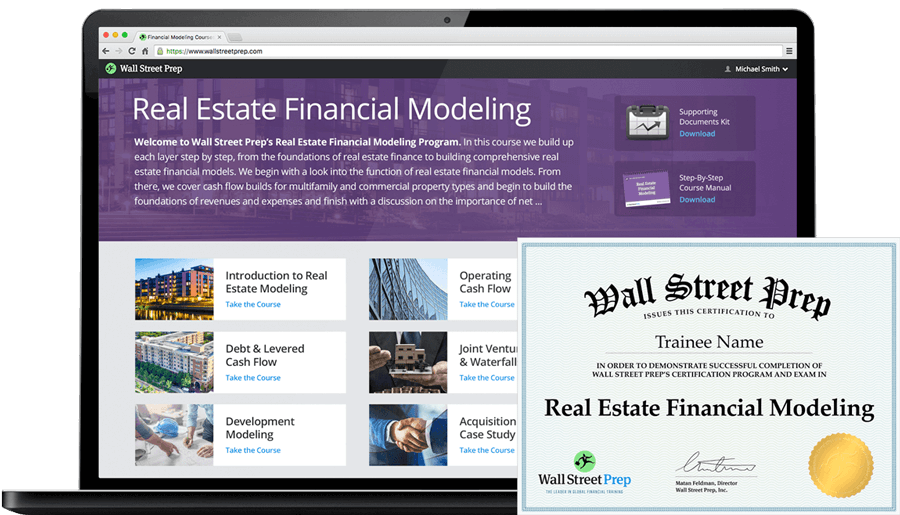20+ Hours of Online Video Training

### Master Real Estate Financial Modeling

This program breaks down everything you need to build and interpret real estate finance models. Used at the world's leading real estate private equity firms and academic institutions.

Inline Feedbacks